Product: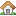Genetic calculator Version: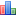4.0 final File size: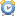14.3 Mb Language:En / Ru License: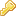Free OS: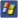Win XP/Vista/7/8

## How to solve polygenic inheritance problems

### Polygenic traits

For some genetic traits is typical a discrete variation - usually, on the basis of such traits, the individuals can be divided into several clearly visible groups. These such traits, that Mendel studied in his experiments, as well as many other traits, which we study in classical genetics in cases of linkage and X-linkage inheritance, as well as the interaction of genes, such as incomplete dominance, codominance or epistasis. For example, the inheritance of hemophilia and blood groups in human, forms of a chicken's combs, the eye color of the fruit fly. Such traits are often called as qualitative, because there are a big difference on these traits between the individuals and it is can be easily observed with a simple look. However, for many other genetic traits is typical the indiscrete variation - these traits more or less indiscrete vary within a certain range, which can be displayed schematically as a bell-shaped curve. These traits include, for example, height of plant, weight of animals, egg laying of chickens, length fibers of flax, sugar in sugar beet roots, the amount of seed corn on the cob, and many others. In human, such traits include for example height, body shape, skin color. Such traits are called quantitative and in contrast with qualitative, the difference on these traits between the individuals is small and requires accurate measurements.

### Polygenic  inheritance

In the early twentieth century, there are was a question to the scientists - how the quantitative traits are inherited. Some of them believed that the inheritance of quantitative differs significantly from the inheritance of qualitative traits, while others believed that the mechanisms of inheritance in both cases are similar in nature. As a result of further experiments was proved, that the last point of view is correct. It turned out, that a indiscrete variation of trait is due to the action of several genes and each of them has a small effect, and is inherited according to Mendel's laws. Such genes was named - polygenes. Obviously, that the more loci are involved and have a cumulative effect on the trait, then variation of this trait will be closer to the indiscrete.

Usually, the action of polygenes has the additive and cumulative character. However, not always all alleles have the same effect on the expression of the trait. Because of the dominance or allelic interaction, the effects of some alleles may be greater than the effect of others. Very often the expression of most quantitative traits depends on the influence of the environment. For example, such environmental factors as diet, climate, disease may have a significant effect on a human's weight, milk yield of cows or the size of corn cob. Therefore, in many cases it is difficult to determine the number of genes, that formed the quantitative trait, by the phenotypic ratio in the offspring.

### Polygenic inheritance examples

In practice, the number of polygenes is often determined by the proportion of individuals in the progeny of the second generation, who have parental phenotype. Perhaps, it's necessary to explain in more detail how this is done. You can see the field with entries "Unique" and "Total" on the right side of the genetic calculator. The entry "Unique" is the number of unique genotypes or phenotypes in the results of crossing, and "Total" the total number of genotypes. To calculate the total number of genotypes can be used very simple formula Total = 2 ^ (2 * number of hybrid genes). For example, for monohybrid crosses Total = 2 ^ (2 * 1) = 4, for dihybrid Total = 2 ^ (2 * 2) = 16, for trihybrid Total = 2 ^ (2 * 3) = 64 and so on. For polygenic crosses, the proportion of dominant or recessive homozygous individuals in the second generation, which will has the parent genotype, is equal to 1/Total. If the formation of the trait is controled a single gene, the proportion of homozygotes individuals = 1/4, if two polygenes, the proportion = 1/16 if the three polygenes, the proportion = 1/64 and so on. Thus if we know the proportion of such recessive homozygotes individuals, then we can determine the number of polygenes that control the trait. Let's look at an example.

#### Color of caryopsis of wheat.

Color of caryopsis of wheat may be of various range of red - from white to a dark-red. All first-generation offspring from crosses between the white and light-red sorts had intermediate color, while in the second generation, the ratio of phenotypes of white, intermediate, and light-red was 1:2:1. In the first offspring generation from crosses of white and red sorts was light-red, while in the second generation could be identify 5 different types coloring of caryopsis - from white to red. The proportion of white caryopsis was approximately equal to 1/16. Hybrids of first-generation from crosses between the white and dark-red sorts had intermediate color of caryopsis, in contrast with their parents, while in the second generation could be identify 7 different types coloring of caryopsis - from white to dark-red. The proportion of white caryopsis was approximately equal to 1/64 from the total amount. So we can conclude that the color of wheat ears is controlled by three genes, each of which has a small effect. Each gene is represented by two alleles, one of which determines the red color and the other white. Let's mark the alleles of these three loci as "Aa", "Bb" and "Cc". Then the genotypes of tese sorts will be as follows: white - "aabbcc", light-red - "AAbbcc", red - "AABBcc" and dark-red - "AABBCC". However, it's necessary to say that the red color of caryopsis will be not only in plants with the genotype "AABBcc", but also in plants with genotypes "AAbbCC", "aaBBCC", "AaBbCC", "AaBBCc", "AABbCc". It is also will be right for light-red plants and plants with intermediate coloration of caryopsis. Let's write the traits file, which will include all the possible variants:

`A A B B C C:dark-red wheatA A B B C:mid dark-red wheatA A B C C:mid dark-red wheatA B B C C:mid dark-red wheatA A B B:red wheatA A C C:red wheatA A B C:red wheatA B B C:red wheatA B C C:red wheatB B C C:red wheatA A B:mid red wheatA A C:mid red wheatA B B:mid red wheatA B C:mid red wheatA C C:mid red wheatB B C:mid red wheatB C C:mid red wheatA A:light-red wheatA B:light-red wheatA C:light-red wheatB B:light-red wheatB C:light-red wheatC C:light-red wheatA:mid light-red wheatB:mid light-red wheatC:mid light-red wheat*:white wheat{color:A acolor:B bcolor:C c}`

Genetic calculation: You can open this file ( Polygenic 1A.txt ) and calculate results for Traits phenotypes.

Parental genotypes for crosses between the white and light-red will be - "aabbcc" for white, and "AAbbcc" or "aaBBcc" or "aabbCC" for a light-red. All first-generation offspring will be mid light-red wheat (for example "Aabbcc"). As a result of crossing first-generation hybrids, we obtain the Traits phenotypes ratio 1:2:1.

Parental genotypes for crosses between the white and red will be - "aabbcc" for white, and "AABBcc" or "aaBBCC" or "AAbbCC" for a light-red. All first-generation offspring will be light-red wheat (for example "AaBbcc"). As a result of crossing first-generation hybrids, we obtain the Traits phenotypes ratio 1:4:6:4:1.

Parental genotypes for crosses between the white and dark-red will be - "aabbcc" for white, and "AABBCC" for dark-red. All first-generation offspring will be mid red wheat ("AaBbÑc"). As a result of crossing first-generation hybrids, we obtain the Traits phenotypes ratio 1:6:15:20:15:6:1.

Traits file in this case look like plenty bulky and complicated. But we can simplify it. Since each dominant allele has the same effect in the exprression of red color, then the color is caused only by the number of dominant alleles and is independent of the locus to which they belong. So we can mark all the polygenes by the same letters, for example "Aa". Then the parental genotypes of wheat with white caryopsis will be "aaaaaa", with a light-red "AAaaaa" or "aaAAaa" or "aaaaAA", with red "AAAAaa" or "aaAAAA" or "AAaaAA", and with dark-red "AAAAAA". And the traits file will look much simpler:

`A A A A A A:dark-red wheatA A A A A:mid dark-red wheatA A A A:red wheatA A A:mid red wheatA A:lighte-red wheatA:mid lighte-red wheat*: white wheat{color:A acolor:A acolor:A a}`

You can open this file ( Polygenic 1B.txt ) and calculate results for Traits phenotypes.

You can get the correct results polygenic crosses without using traits files. The fact that in the case of polygenic inheritance ratio of Traits phenotypes equivalent to a simple ratio of genotypes. If the dominant and recessive alleles of polygenes are marked by the same letters, but in a different register (dominant - in the upper case and the recessive in lower case), then starting with version 3.3 you can write the parental genotypes without using special characters. But if you mark a dominant alleles with different letters, then each group of polygenes in the parental genotypes entry, you must conclude in the colons (":") characters. Choose the item "Genotypes" and you will see, that by crossing of first generation hybrids we obtain the ratio 1:2:1 for the hybrids with the genotype "Aaaaaa", 1:4:6:4:1 for "AaAaaa" and 1:6:15:20:15:6:1 for "AaAaAa". You can also combine this method of solving genetic problems with using of genetic traits files.

#### Length of the ears of rabbits

Let's look at another example. Suppose, that the length of the ears of rabbits is controlled by two pairs of polygenes. Dominant alleles of these loci we mark as "D", and the recessive alleles as "d". The length of the ears of dominant homozygous rabbits with genotype "DDDD" is equal to 28 centimeters, and the length of the ears of recessive homozygous rabbits with genotype "dddd" is equal to 12 centimeters. If four recessive alleles gives us 12 centimeters of length, then one allele gives us 3 centimeters (12/4 = 3). And one dominant allele gives us 7 centimeters (28/4 = 7). Thus we can say that each dominant allele increases the length of the ears by 4 centimeters (7 - 3 = 4). Then the length of the ears of rabbits with three dominant alleles will be equal to 24 centimeters (28 - 4 = 24), with two - 20 centimeters (24 - 4 = 20), and with one will be equal to 16 centimeters (20 - 4 = 16). And we can write our traits file like this:

`D D D D:length28D D D:length24D D:length20D:length16*:length12 {length:D dlength:D d}`

Genetic calculation: You can open this file ( Polygenic 2A.txt ) and calculate results for Traits phenotypes. If you cross rabbits with genotypes "DDDD" and "dddd", then all the hybrids of first generation will have a length of ears equal to 20 centimeters ("DdDd"). As result of crossing the hybrids of first generation we get the offspring with Traits phenotypes ratio: 1:4:6:4:1.

Since we are dealing with quantitative traits, we can mark traits in the traits file only as a numerical values. In this case, the program independently calculate the length of ears for each genotype in the offspring and give us the correct ratio of Traits phenotypes. This can be done in two ways. We know that a dominant allele gives us 7 centimeters, and a recessive allele gives us 3 centimeters. Then we will form the traits file like this:

`D:7d:3{length:D dlength:D d}`

And it's can be done in another way. The length for genotype "dddd" is 12 centimeters. We take it as a base value for any genotype and for marking this value we use the symbol "*". Each dominant allele increases the length by 4 centimeters. This value will be added to the base value. Then we will form the traits file like this:

`D:4*:12{length:D dlength:D d}`

You can open this files ( Polygenic 2B.txt or Polygenic 2C.txt ) and calculate results for Traits phenotypes. If you cross the rabbits of first-generation hybrids ("DdDd"), you will see the same ratio of Traits phenotypes - 1:4:6:4:1.

And of course as well as in the previous example, you can solve this problem using the last method. Choose the item "Genotypes" and you will see that by crossing the first generation hybrids we obtain the same ratio. You can also combine this method of solving genetic problems with using of genetic traits files.

#### Downiness of rye of caulis.

Consider another example. As result of study of several thousand plants of rye was found, that the downiness of the caulis in plants is highly varies - from 60 fibrils per square centimeter to the complete lack of downiness. It turned out that this trait is controlled by three polygenes. Let us mark a dominant allele of this gene as "B", and recessive allele as "b". And I want to draw your attention to the following condition: each dominant gene in the homozygous or heterozygous state gives us downiness equal to 20 fibrils per square centimeter of the caulis. What does this mean? This means that plants with the genotype "BB" and with the genotype "Bb" would have the identical phenotype. If in the above examples, the ratio of Traits phenotypes was be equal to the simple genotypes ratio, then in this case it is not. In this example, the ratio of Traits phenotypes would be equivalent to a simple ratio of phenotypes. Therefore, to get correct results, we must check the checkbox "From Phenotypes" in the program (if you do not, you will get the wrong answer.) Thus the ratio of Traits phenotypes will be formed by the phenotypic ratio. This condition, we must take into account when we write Traits file. For example, plants with genotypes "BBbbbb" and "Bbbbbb" will have downiness equal to 20 fibrils, plants with the genotypes "BBBBbb" and "BbBbbb" equal to 40 fibrils, and plants with the genotypes "BBBBBB" and "BbBbBb" equal to 60 fibrils. Thus, a dominant allele in the Traits file notation will symbolize the presence of one or two dominant alleles at one locus. And our Traits file would look like:

`B B B:downiness60B B:downiness40B:downiness20b:without downiness{downiness:B bdowniness:B bdowniness:B b}`

As in the previous example, we can mark traits in the traits file as numeric values. Necessary to clarify, that a trait of lack of downiness, we must leave as string value. Of course, we can mark this trait as 0 (zero) and the program will give the correct ratio, but the phenotype notation will be not correct (unknown). Therefore, in this case, we can combine numeric and string values ??and get the correct result. Our Traits file would look like:

`B:20*:without downiness{downiness:B bdowniness:B bdowniness:B b}`

Genetic calculation: You can open this files ( Polygenic 3A.txt or Polygenic 3B.txt ) and calculate results for Traits phenotypes. If you cross hybrid plants with the genotypes "BbBbBb", then as result we can get a Traits phenotypes ratio 27:27:9:1. And of course you can solve this problem using a last method. Only in this case you need choose not the item "Genotypes, but the item "Phenotypes". You will see the same ratio. You can also combine this method of solving genetic problems with using of genetic traits files.

#### Length of spike of barley rachis.

When we can not under the terms of the genetic problems to use the same letters for polygenes, then marking traits as numeric values can greatly facilitate the creation of our traits file. Consider this on example of the length of barley rachis. Rachis of barley consists of segments. In these segments are arranged spikelets. The density and shape of the spike depends on the length of each segment - the shorter it is, the denser spike. This trait is controlled by four polygenes. We mark them as "A", "B", "C" and "D". In homozygous recessive line with genotype "aabbccdd" spike very dense. The length of one segment is equal to 1.15 millimeters. In the homozygous dominant line with genotype "AABBCCDD" the spike is loose and segments is long. The length of one segment is equal to 3.33 millimeters. The dominant allele of a gene "A" in the homozygous or heterozygous state increases the length of the segment compared to the recessive on the 0.16 millimeters, "B" on the 0.27 millimeters, "C" on the 0.82 millimeter, "D" on the 0.93 millimeter. In this case, the genes have different effects on the trait and we can't mark them by the same letter. Thus, for example, the length for genotype "AABBCCDD" is the sum of the base value of 1.15 and additional values ??of all dominant alleles, ie 1.15 + 0.16 + 0.27 + 0.82 + 0.93 = 3.33. As the base value for any genotype, we take the length of the segment for the homozygous recessive line with genotype "aabbccdd". Now let's write our traits file. For that he was not so bulky and complex as in the first example, we mark the traits as numerical values:

`A:0.16B:0.27C:0.82D:0.93*:1.15{length:A alength:B blength:C clength:D d}`

Genetic calculation: You can open this file ( Polygenic 4.txt ) and calculate results for Traits phenotypes. In this case, as in the previous example, plants with the genotype "AA" and genotype "Aa" will have the identical phenotype. Therefore, to get correct results, we must check the checkbox "From Phenotypes" in the program. If you cross hybrid plants with the genotype "BbBbBb", as a result we get the Traits phenotypes ratio - 81:27:27:27:27:18:9:9:9:9:3:3:3:3:1. This ratio is very similar to the ratio of genotypes 81:27:27:27:27:9:9:9:9:9:9:3:3:3:3:1, but in this case, plants with genotypes "A_bbccD_" and "aaB_C_dd" have a same phenotype. Therefore, in this case, the Traits phenotypes ratio does not equal to the genotypic ratio and to the phenotypic ratio.

In various sources is not paid very much attention to polygenic inheritance. This is probably due to some difficulties in studying of polygenes. Meanwhile, polygenic inheritance is quite an important subject in genetics. Particularly very important role this type of inheritance have in plant and animals breeding, because many traits of agricultural plants and animals are controlled by polygenes. Breeders are trying to breed cows with the better yield of milk, hens with better qualities of egg-laying or sorts of corn with a large number of seeds in the cob. I hope, that the opportunities provided by a genetic calculator to work with polygenes will greatly facilitate to you the solving of practical problems associated with polygenic inheritance.